Courses

# Test: Gas Turbine Power Plants - 3

## 10 Questions MCQ Test Topicwise Question Bank for Mechanical Engineering | Test: Gas Turbine Power Plants - 3

Description
This mock test of Test: Gas Turbine Power Plants - 3 for Mechanical Engineering helps you for every Mechanical Engineering entrance exam. This contains 10 Multiple Choice Questions for Mechanical Engineering Test: Gas Turbine Power Plants - 3 (mcq) to study with solutions a complete question bank. The solved questions answers in this Test: Gas Turbine Power Plants - 3 quiz give you a good mix of easy questions and tough questions. Mechanical Engineering students definitely take this Test: Gas Turbine Power Plants - 3 exercise for a better result in the exam. You can find other Test: Gas Turbine Power Plants - 3 extra questions, long questions & short questions for Mechanical Engineering on EduRev as well by searching above.
QUESTION: 1

### A gas turbine operates between 5°C and 839°. What is the pressure ratio at which maximum work is obtained?

Solution:

For a simple cycle,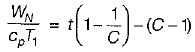for maximum work,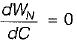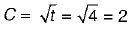∴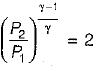or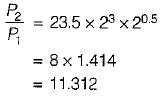QUESTION: 2

### A gas turbine works on which one of the following cycles?

Solution:

Gas turbines works on the Brayton cycle.

QUESTION: 3

### Brayton cycle with infinite intercooling and reheating stages would approximate a

Solution:

Brayton cycle with infinite intercooling and reheating stages would approach the Ericsson cycle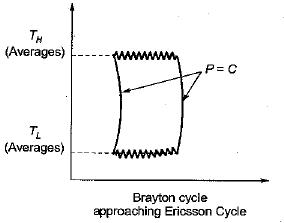QUESTION: 4

Which one of the following additions/sets of additions simple gas turbine cycle will have NO effect on the power output the cycle?

Solution:

Since the exhaust gases of the gas turbine having very high pressure and temperature, these gases are used to heat the air before going to the combustion chamber of gas turbine which reduces the overall heat addition in the combustion chamber, But workout put will be same as without regeneration.

QUESTION: 5

In a gas turbine, working on Brayton cycle with regeneration, air exits from the compressor at 550 K and gas exits from the turbine at 800 K. If the effectiveness of the regenerator is 0.8, then what is the air temperature at the combustion chamber inlet?

Solution: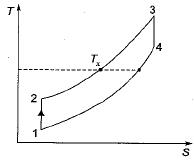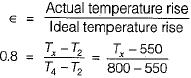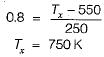QUESTION: 6

A gas turbine power plant has a specific output of 350 kJ/kg and an efficiency of 34%. A regenerator is installed and the efficiency increases to 51%. The specific output wil! be closest to

Solution:

In case of gas turbine, regeneration decreases the heat supplied. It has no effect on power output.

QUESTION: 7

The given figure shows an open-cycle gas turbine employing reheating, on T-S plane. Assuming that the specific heats are same for both air and gas, and neglecting the increase in mass flow due to addition of fuel, the efficiency is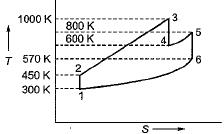Solution:

WT = Cp(T3 - T4) + Cp(T5 - T6)
= Cp(1000 - 600) + Cp(800 - 570)
= 400Cp + 230Cp = 630Cp
WC = Cp(T2 - T1)
= Cp(450 - 300) = 150Cp kJ/kg
Heat supplied
= Cp(T3 - T2) + Cp(T5 - T4)
= Cp(1000 - 450) + Cp(800 - 600)
= 550Cp + 200Cp = 750Cp kJ/kg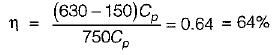QUESTION: 8

The cycle shown in the given figure represents a Gas Turbine Cycle with inter-cooling and reheating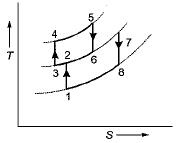Match List-l (Units) with List-II (Processes) and select the correct answer using the codes given below the lists:
List-l
A. Intercooler
B. Combustor
C. Reheater
D. High pressure compressor
List-lI
1. 1-2
2. 2-3
3. 3-4
4. 4-5
5. 6-7
Codes:
A B C D
(a) 1 2 4  3
(b) 2 4 5  3
(c) 3 4 5  2
(d) 2 4 5  1

Solution:
QUESTION: 9

Which one of the following is the correct statement?
In a two-stage gas turbine plant with inter-cooling and reheating

Solution:
QUESTION: 10

In a two-stage gas turbine plant operating on a Brayton cycle with regeneration,

Solution: